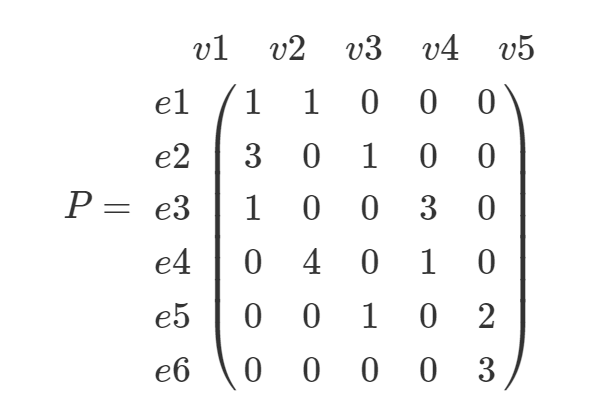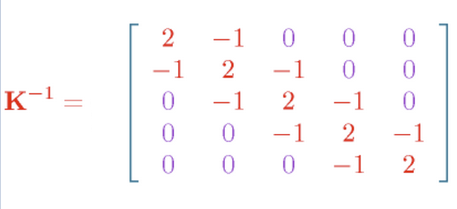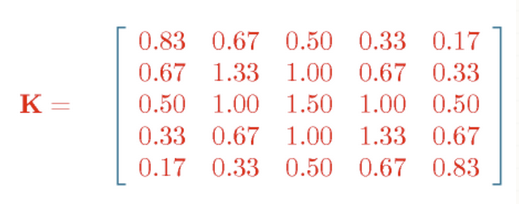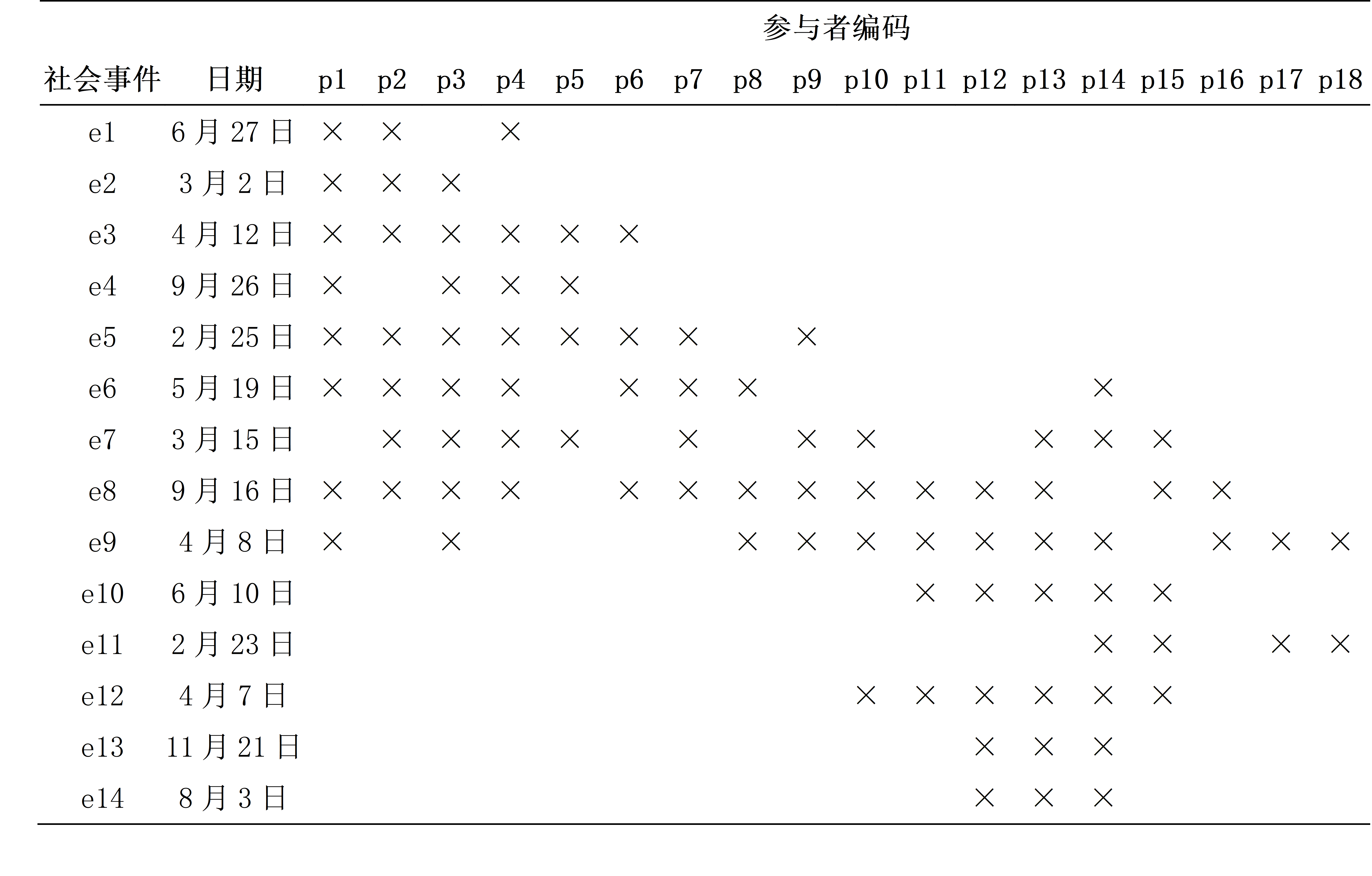# 导言# 高斯图模型

## 高斯图模型的基本形式

$$X = (X_{1},...,X_{p}) \sim N(\mu,\Sigma)\backslash n$$$\Theta$矩阵与偏相关系数有如下关系：

$$\rho_{ij \mid { i,j}} = - \frac{\omega_{\text{ij}}}{\sqrt{\omega_{\text{ii}}\omega_{\text{jj}}}}$$

$$\text{log\ }\text{det\ Θ} - trace(\text{SΘ})$$ （1）

## 罚似然估计法

### 罚似然估计法

$$\text{maximiz}e_{\Theta}{ log\text{detΘ} - trace(\text{SΘ}) - \lambda \mid \mid \Theta \mid \mid_{1}}$$

$$RSS=\sum_{i=1}^{n}(y_i - \beta_0 - \sum_{j=1}^{p}\beta_jx_{ij})^2$$

$$RSS + \lambda \sum_{j=1}^{p} |(\beta_j)|$$

## 应用与示例# DGG 数据及示例

## 数据载入
library(igraph)
dgg[is.na(dgg)]<-0
dgg<-t(dgg[,-1])
colnames(dgg)<-c(1:18)
dim(dgg)
dgg

## 基本 glasso 模型

library(glasso)

result = glasso(cov(dgg), rho = 0.1, penalize.diagonal=F)

# 计算偏相关系数矩阵
wi_to_pcor <- function(wi) {
p = -wi
d = 1/sqrt(diag(wi))
pcor = diag(d)%*%p%*%diag(d)
diag(pcor) = 0
colnames(pcor) <- seq_len(ncol(pcor))
pcor
}

pcor = wi_to_pcor(result$wi) # 偏相关系数矩阵 pcor g = graph.adjacency(pcor > 0, mode="undirected", diag = FALSE) plot(g, vertex.color = 'white', vertex.size = 20, edge.color = 'black') rhos <- c(0.05, 0.08, 0.1, 0.2, 0.8) ret <- lapply(rhos, glasso, s = cov(dgg), penalize.diagonal = F) wi <- lapply(ret, getElement, 'wi') ret.pcor <- lapply(wi, wi_to_pcor) ret.mat <- lapply(ret.pcor, function(x) x > 0) ret.g <- lapply(ret.mat, graph.adjacency, mode="undirected", diag = FALSE) for (i in seq_along(ret.g)) { plot(ret.g[[i]], vertex.color = 'white', vertex.size = 20, edge.color = 'black', main = sprintf("rho = %.2f", rhos[i])) } #' @param x list of glasso result get_ebic <- function(x, n, gamma = 0.01) { df <- (sum(abs(x$wi) > 1e-5) - ncol(x$wi)) / 2 d <- ncol(x$wi)
-2 * x\$loglik + df * log(n) + 4 * df * gamma * log(d)
}
ebic <- sapply(ret, get_ebic, n = 14, gamma = 0.1)
plot(rhos[1:4], ebic[1:4], type='l', xlab='rho', ylab='eBIC')